Sums an array of numbers log(x1)...log(xn) : Array « Collections Data Structure « Java

Sums an array of numbers log(x1)...log(xn)

```
/* Copyright (C) 2003 Univ. of Massachusetts Amherst, Computer Science Dept.
This file is part of "MALLET" (MAchine Learning for LanguagE Toolkit).
http://www.cs.umass.edu/~mccallum/mallet
This software is provided under the terms of the Common Public License,
information, see the file `LICENSE' included with this distribution. */

//package cc.mallet.util;

/**
*
*
* @author <a href="mailto:casutton@cs.umass.edu">Charles Sutton</a>
* @version \$Id: ArrayUtils.java,v 1.1 2007/10/22 21:37:40 mccallum Exp \$
*/
public class Util {
private static final double LOGTOLERANCE = 30.0;
/**
* Sums an array of numbers log(x1)...log(xn).  This saves some of
* <p>
* Note that this implementation IGNORES elements of the input
*  array that are more than LOGTOLERANCE (currently 30.0) less
*  than the maximum element.
* <p>
* Cursory testing makes me wonder if this is actually much faster than
*  repeated use of the 2-argument version, however -cas.
* @param vals An array log(x1), log(x2), ..., log(xn)
* @return log(x1+x2+...+xn)
*/
public static double sumLogProb (double[] vals)
{
double max = Double.NEGATIVE_INFINITY;
int len = vals.length;
int maxidx = 0;

for (int i = 0; i < len; i++) {
if (vals[i] > max) {
max = vals[i];
maxidx = i;
}
}

double intermediate = 0.0;
double cutoff = max - LOGTOLERANCE;

for (int i = 0; i < maxidx; i++) {
if (vals[i] >= cutoff) {
intermediate += Math.exp(vals[i] - max);
}
}
for (int i = maxidx + 1; i < len; i++) {
if (vals[i] >= cutoff) {
intermediate += Math.exp(vals[i] - max);
}
}

return max + Math.log(1.0 + intermediate);
} else {
return max;
}

}
}

```

Related examples in the same category

 1 Initialize a static array 2 Initialization and re-assignment of arrays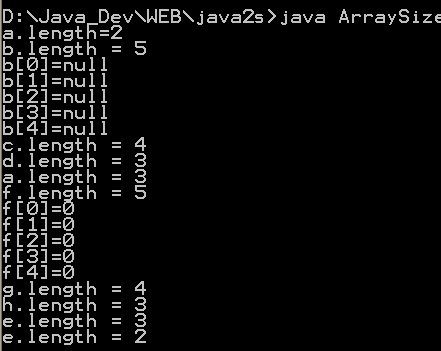3 Doubling the size of an array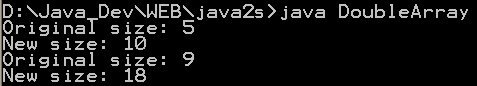4 Timing array loop performance 5 Array 2D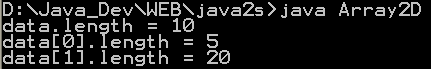6 Can you change the .length of an array 7 Show Two-Dimensional Array of Objects 8 ArrayListDemo done over using an ArrayList 9 Array Hunt game 10 Multi Dimension Array 11 Clone Array 12 Associates keys with values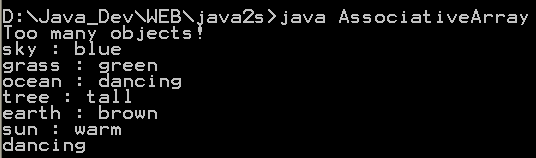13 Arrays of primitives 14 Creating arrays with new 15 Array initialization 16 Creating an array of nonprimitive objects 17 Create multidimension arrays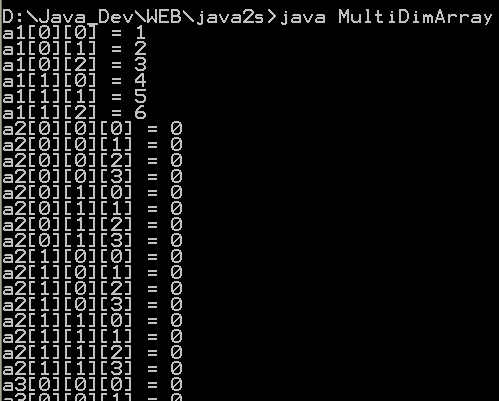18 Initializing Array Values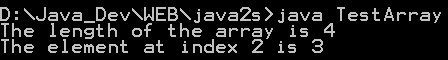19 Creating a Two-Dimensional Array 20 Initializing a Two Dimensional Array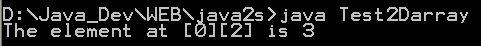21 Using the length Variable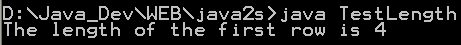22 Triangular array 23 Grow array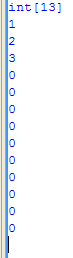24 Define array for class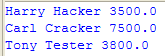25 String array and output to console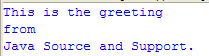26 Multiply two matrices 27 Array Of Arrays Demo 2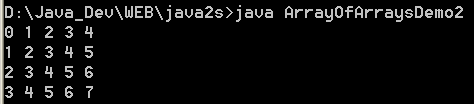28 Array Copy Demo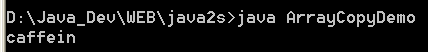29 Copying Elements from One Array to Another 30 Java program to demonstrate multidimensional arrays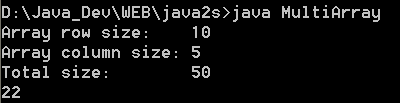31 Extend the size of an array 32 Copy an array 33 Initialize multidimensional array 34 Get array upperbound 35 To get the number of dimensions 36 Resize an array, System.arraycopy() 37 Dump array content: Convert the array to a List and then convert to String 38 java.utils.Arrays provides ways to dump the content of an array. 39 Dump multi-dimensional arrays 40 Use the new shorthand notation to iterate through an array 41 Create a repeated sequence of character 42 Reverse array elements order 43 Convert array of primitives into array of objects 44 Array Initializers 45 Reinitializes a byte array 46 Reinitializes an int array 47 Sum all elements in the array 48 A class to iterate over all permutations of an array. 49 Palidrome Array 50 Set of utilities used to manipulate arrays. 51 ArrayUtils provides static methods for manipulating arrays when using a tool such as java.util.ArrayList is inconvenient. 52 Array Util 53 clone two dimensional array